# Current Electricity How do we describe current electricity

• Slides: 28Current Electricity How do we describe current electricity?Electric Current • The flow of electrons through a conductor.Electric Current Examples • • • Electric hair straightner. Hair dryer Microwave Cell phone Cell chargerConductor • A material that easily allows electrons to flow through it.Conductor Examples • Copper wire • All metals • Water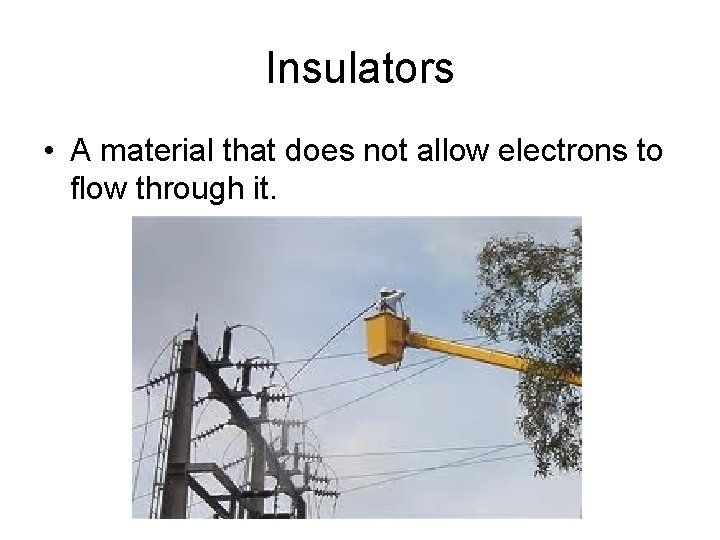Insulators • A material that does not allow electrons to flow through it.Insulators Example • • • Plastic Rubber Glass Wax AirAlternating Current • The electrons alternate or change direction of flow. The abbreviation is AC.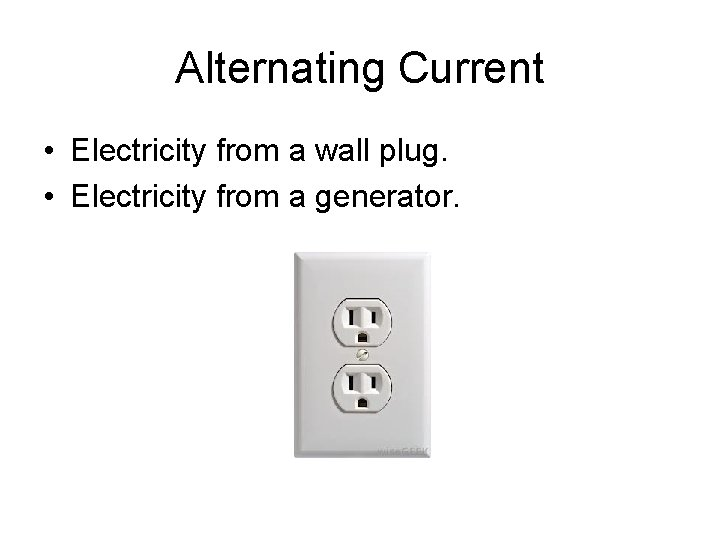Alternating Current • Electricity from a wall plug. • Electricity from a generator.Direct Current • The electrons flow in one direction. The abbreviation is DC.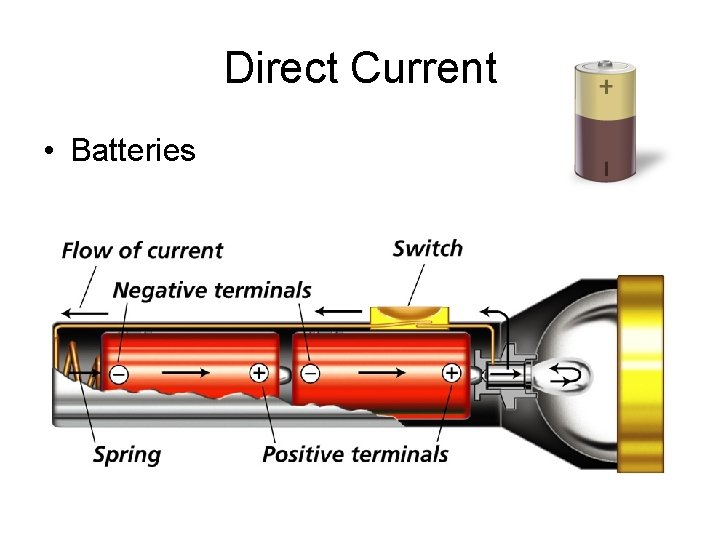Direct Current • Batteries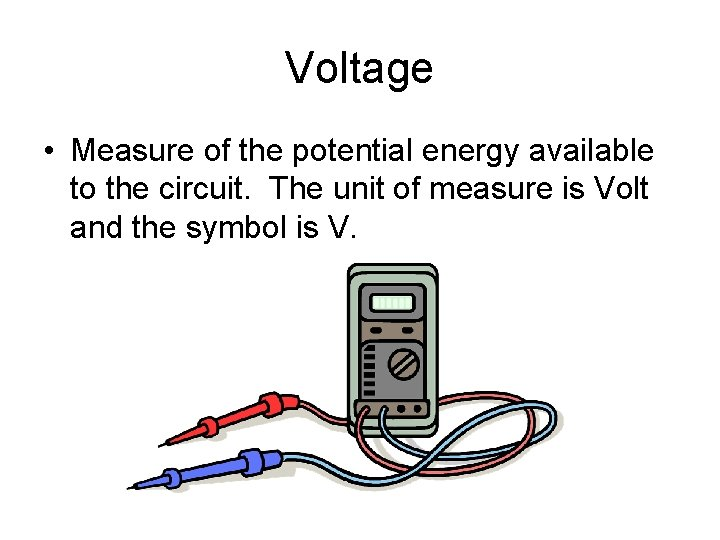Voltage • Measure of the potential energy available to the circuit. The unit of measure is Volt and the symbol is V.Voltage • Car battery – 12 volts • D cell battery – 1. 5 volts • House circuit – 120 volts except for the clothes dryer = 240 volts.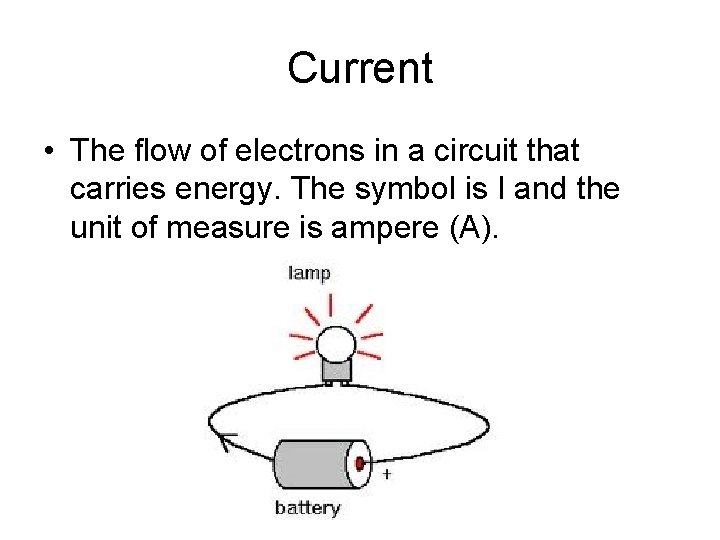Current • The flow of electrons in a circuit that carries energy. The symbol is I and the unit of measure is ampere (A).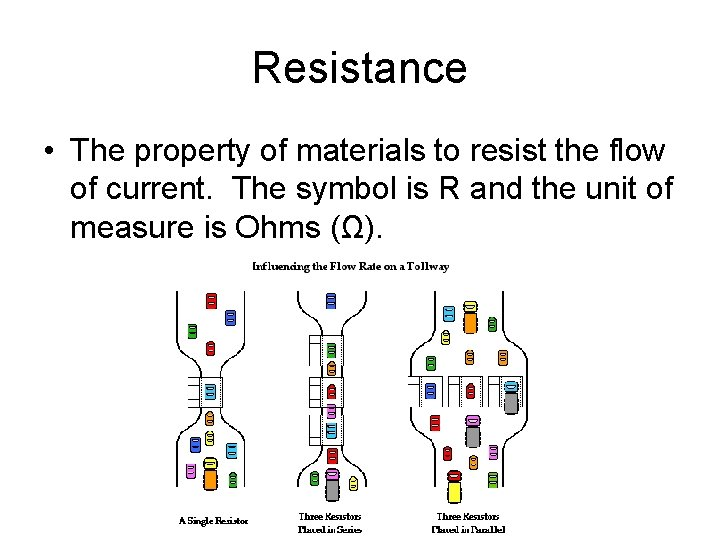Resistance • The property of materials to resist the flow of current. The symbol is R and the unit of measure is Ohms (Ω).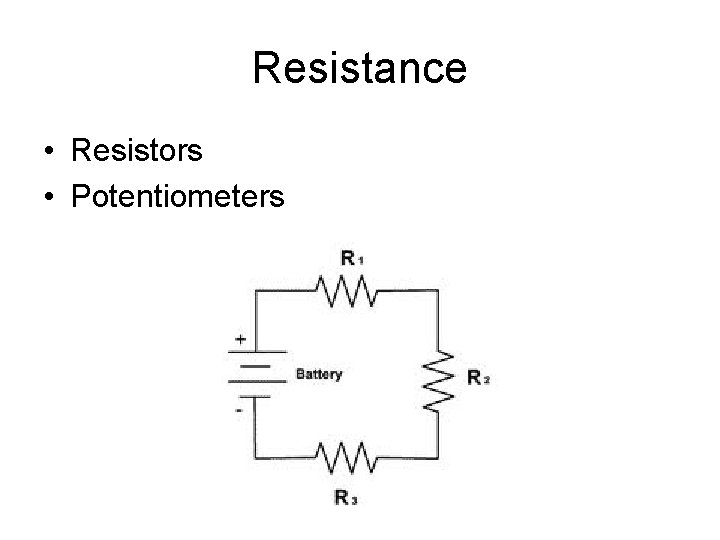Resistance • Resistors • Potentiometers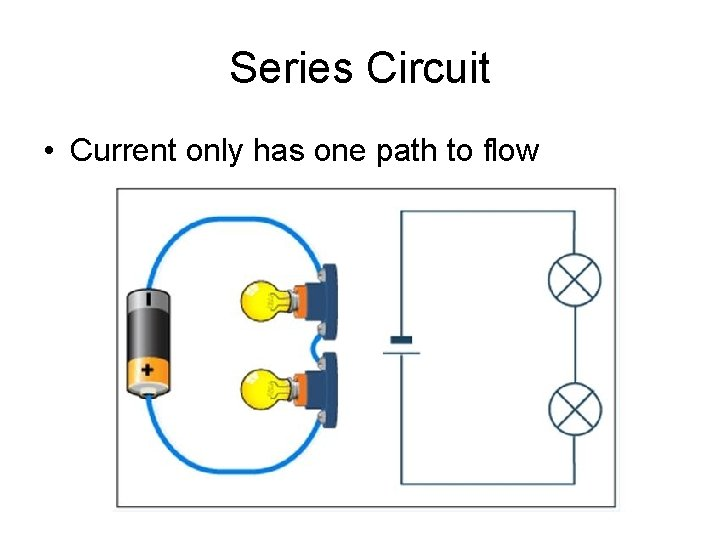Series Circuit • Current only has one path to flow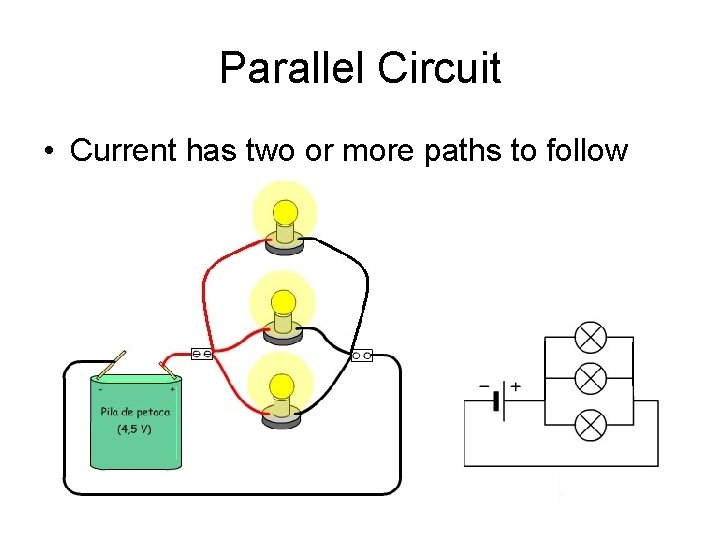Parallel Circuit • Current has two or more paths to follow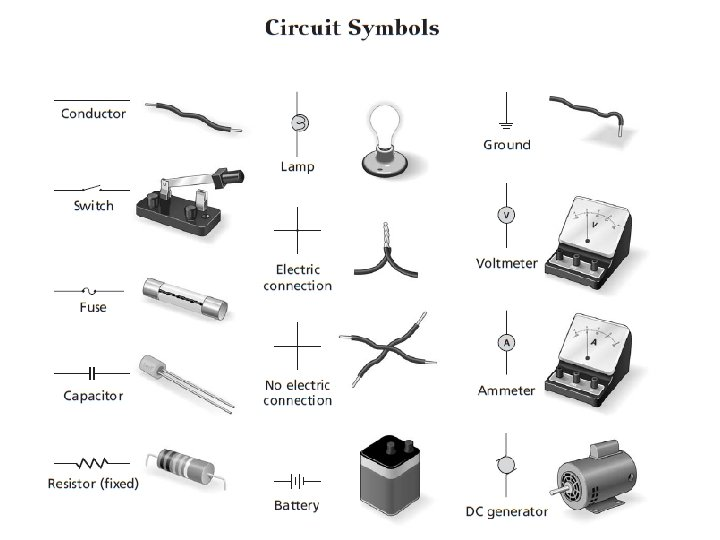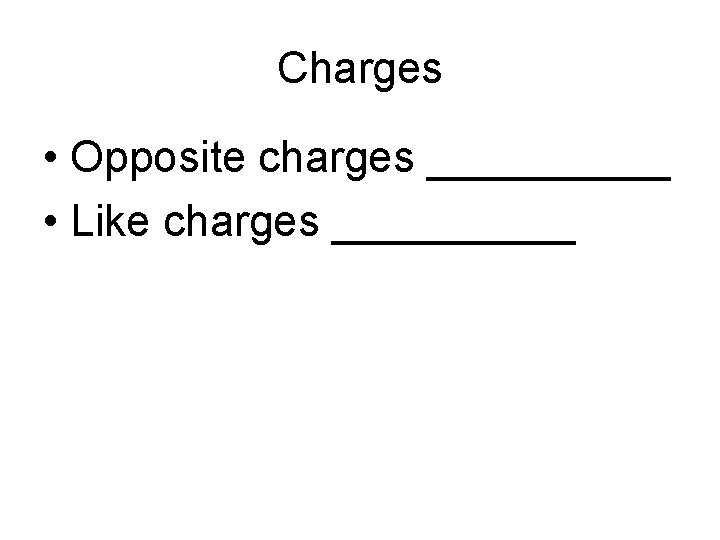Charges • Opposite charges _____ • Like charges _____Charges • If Balloon B is Negative • Balloon A is ____ • Balloon C is ____Charges • If Balloon B is negative • Balloon A is _____ • Balloon C is _____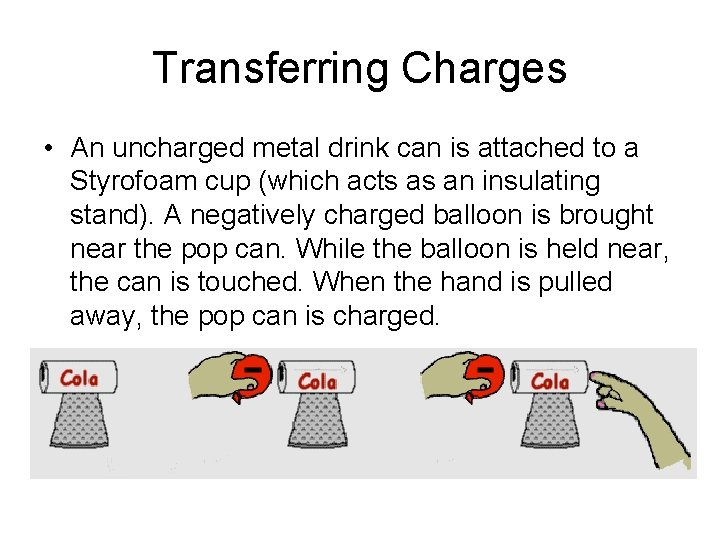Transferring Charges • An uncharged metal drink can is attached to a Styrofoam cup (which acts as an insulating stand). A negatively charged balloon is brought near the pop can. While the balloon is held near, the can is touched. When the hand is pulled away, the pop can is charged.Transferring Charges • This process is known as charging by_____.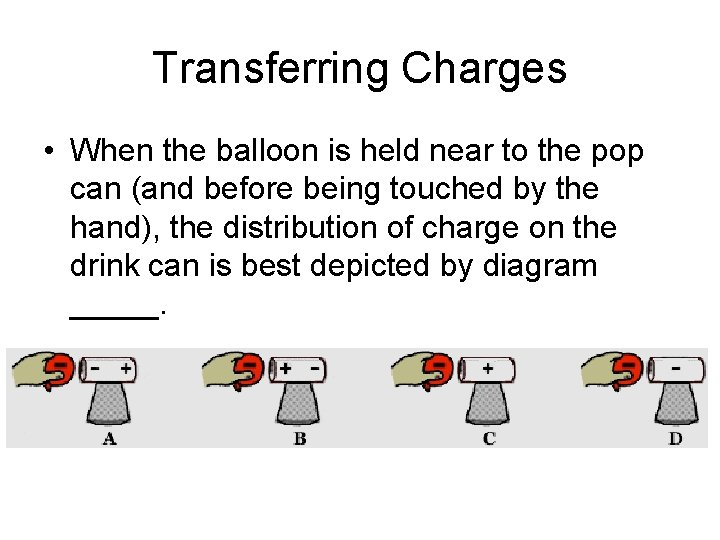Transferring Charges • When the balloon is held near to the pop can (and before being touched by the hand), the distribution of charge on the drink can is best depicted by diagram _____.Transferring Charges • When the drink can is touched by the hand, ______ move from the ______ to the _______. a. protons, hand, can b. protons, can, hand c. electrons, hand, can d. electrons, can, hand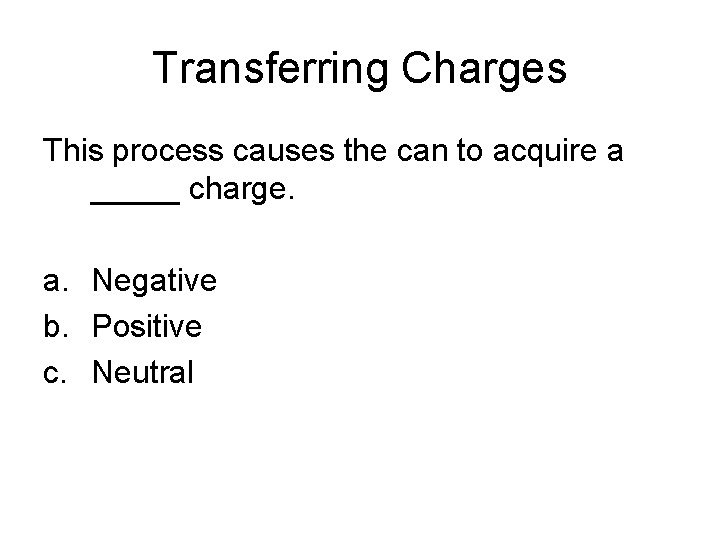Transferring Charges This process causes the can to acquire a _____ charge. a. Negative b. Positive c. Neutral